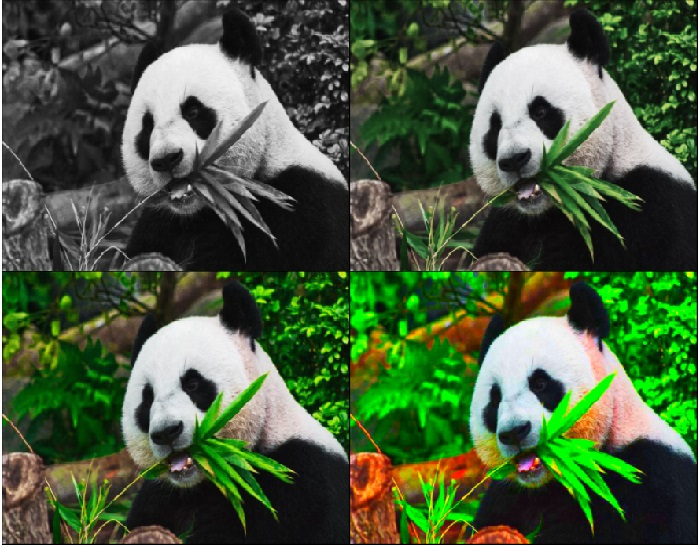# How to adjust saturation of an image in PyTorch?

The saturation of an image refers to the intensity of a color. The higher the saturation of a color, the more vivid it is. The lower the saturation of a color, the closer it is to gray.

To adjust the saturation of an image, we apply adjust_saturation(). It's one of the functional transforms provided by the torchvision.transforms module. adjust_saturation() transformation accepts both PIL and tensor images. A tensor image is a PyTorch tensor with shape [C, H, W], where C is the number of channels, H is the image height, and W is the image width.

This transform also accepts a batch of tensor images. If the image is neither a PIL image nor a tensor image, then we first convert it to a tensor image and then apply adjust_saturation(). The saturation value should be a non-negative number.

## Syntax

torchvision.transforms.functional.adjust_saturation(img, saturation_factor)

## Parameters

• img - Image of which saturation is to be adjusted. It is a PIL image or torch tensor. It may be a single image or a batch of images.

• hue_factor - A non-negative number. 0 will give a black-and-white image, while 1 will give the original image.

## Output

It returns the saturation adjusted image.

## Steps

To adjust the saturation of an image, one could follow the steps given below −

• Import the required libraries. In all the following examples, the required Python libraries are torch, Pillow, and torchvision. Make sure you have already installed them.

import torch
import torchvision
import torchvision.transforms.functional as F
from PIL import Image
• Read the input image. The input image is a PIL image or a torch tensor

img = Image.open('panda.jpg')
• Adjust the saturation of the image with the desired saturation factor.

img = F.adjust_saturation(img, 5)
• Visualize the saturation adjusted image.

img.show()


## Input Images

We will use this image as the input file in the following examples## Example 1

In this program, we adjust the saturation of the input image with saturation_factor=5.

import torch
import torchvision
import torchvision.transforms as T
import torchvision.transforms.functional as F

# convert img1 to PIL image
img1 = T.ToPILImage()(img1)

# display the PIL image
img1.show()

## Output## Example 2

In this program, we adjust the saturation of the input image with different saturation_factor.

import torch
import torchvision
import torchvision.transforms as T
import torchvision.transforms.functional as F
from torchvision.utils import make_grid

# make image grid
grid_img = make_grid([img1, img2, img3, img4], nrow=2)

# convert the tensor image to PIL image
grid_pil = T.ToPILImage()(grid_img)

# display the PIL image grid
grid_pil.show()

## Output